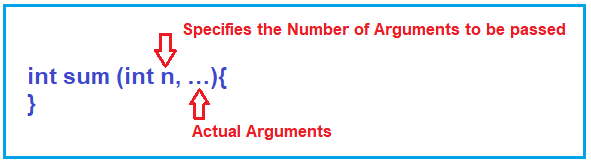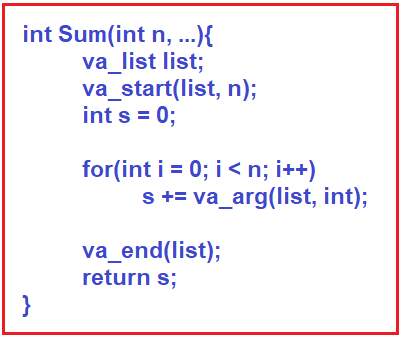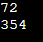# Ellipsis in C++

## Ellipsis in C++ with Examples:

In this article, I am going to discuss Ellipsis in C++ with Examples. Please read our previous article where we discussed InClass Initializer and Delegation of Constructors in C++ with Examples. The Ellipsis in C++ was Introduced in C++ 11.

##### Ellipsis in C++:

Ellipsis is used for taking a variable number of arguments in a function. For example, if we want to write a function for finding the sum of elements or sum of integers then we want our function to work with a different number of parameters like,
Sum (10, 20) = 30
Sum (30, 54, 23) = 107

In this way, we want our function to work with a different number of parameters. We want it just like printf function.
printf (” “, –, –, –)

In printf function, we can pass as many arguments as we want. So just like printf function which takes a variable number of arguments. In the same way, we want our function which works for a variable number of arguments. So, for this, the ellipsis is useful.

##### How to write Ellipsis in C++?

One important thing is the function should know the number of parameters given inside the function then it can work on that many arguments. Suppose we have passed 3 arguments then that function must know how many arguments we have passed.

###### Syntax of Ellipsis:This is the syntax of a function that can take n number of arguments. In the above declaration, n represent the number of arguments the function is taking and 3 dots represent a variable number of arguments. So,

1. If we want to pass 3 numbers then n will be 3 and then we will pass 3 numbers.
2. If we want to pass 10 numbers then n will be 10 and then we will pass 10 numbers.

So, we have to mention the number of arguments that we are passing in the function. The function can get any number of parameters.

So, if we want to send 3 numbers then we will write Sum (3, 3, 4, 6) and if we want to send 10 numbers then we will write Sum (10, 3, 4, 5, …). So first we have to write the number of arguments and then we have to pass all the numbers. So, 3 and 10 will go to the n, and the rest of the numbers will be in 3 dots. Now let us see how to access these numbers inside the function.

##### How to access Elements using Ellipsis in C++?

For accessing the elements, there is a class available in C++ that is va_list. For a better understanding, please have a look at the following code.This is the function for calculating the sum of n integers. First, we have created an object list of the type va_list class. Then we call the function va_start. This function is taking 2 arguments which are the list and the number of arguments. This will start that argument list. It will take all the arguments in the form of a list that we have passed in the function.

Then we have written for loop for accessing all the elements and stored the addition in the s variable. Inside the loop, we have used the va_arg function. This function is taking the list and the data type that is int. This function will be getting all the elements one by one from the list. Every time we have to mention the data type whenever we are getting the elements from the list.

Then we have written va_end (list). This function will end the list of arguments. Then we have returned s which is the sum of elements.

##### What are the important instructions in this function?

va_list(), va_start(), va_arg() and va_end().

In this way, we can access the variable number of arguments. Now let us see the complete program for this one.

##### Example to Understand Ellipsis in C++:
```#include<iostream>
#include<cstdarg>
using namespace std;

int sum (int n, ...)
{
va_list list;
va_start (list, n);
int x;
int s = 0;
for (int i = 0; i < n; i++)
{
x = va_arg (list, int);
s += x;
}
return s;
}

int main()
{
cout << sum (3, 12, 24, 36) << endl;
cout << sum (7, 13, 26, 39, 52, 65, 78, 81) << endl;
}
```
###### Output:In the next article, I am going to discuss Number Systems in C++ with Examples. Here, in this article, I try to explain Ellipsis in C++ with Examples and I hope you enjoy this article. I would like to have your feedback. Please post your feedback, question, or comments about Ellipsis in C++ with the Examples article.

### 1 thought on “Ellipsis in C++”

1.Nice Article and very easy to understand.